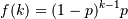# numpy.random.geometric¶

numpy.random.geometric(p, size=None)

Draw samples from the geometric distribution.

Bernoulli trials are experiments with one of two outcomes: success or failure (an example of such an experiment is flipping a coin). The geometric distribution models the number of trials that must be run in order to achieve success. It is therefore supported on the positive integers, k = 1, 2, ....

The probability mass function of the geometric distribution iswhere p is the probability of success of an individual trial.

Parameters : p : float The probability of success of an individual trial. size : tuple of ints Number of values to draw from the distribution. The output is shaped according to size. out : ndarray Samples from the geometric distribution, shaped according to size.

Examples

Draw ten thousand values from the geometric distribution, with the probability of an individual success equal to 0.35:

```>>> z = np.random.geometric(p=0.35, size=10000)
```

How many trials succeeded after a single run?

```>>> (z == 1).sum() / 10000.
0.34889999999999999 #random
```

#### Previous topic

numpy.random.gamma

#### Next topic

numpy.random.gumbel